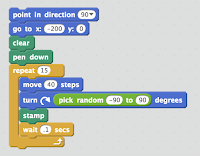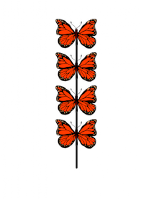## Sunday, January 14, 2018

### Image Integration With the Turtle Library for Processing

In this post I'm going to explore using Leah Beuchley's Turtle library for Processing to "stamp" images in a sort of port of Scratch's ability to stamp a sprite's costume. The turtle's self knowledge of its heading and position will come in handy here, though the way Processing's rotation method works make it a little tricky at points, but certainly worth while.Something to try is creating a graphic that models an animal's movement, such as a butterfly, like I did here with Scratch.
I was reading here that butterflies maneuver turns of up to 90 degrees in the distance of their short bodies! So in Scratch I modeled at least the X-Y trajectory of a butterfly with a range of -90 to 90 degrees of random turning with each step.To set this up in Processing first let's create the turtle and display butterfly image at the turtle's starting location. The turtle instance start location is in the middle of the window (width/2, height/2), so the butterfly will display there when we use the getX() and getY() functions on the turtle instance.
`````` import Turtle.*;
Turtle t;
PImage butterfly;
void setup() {
size(500, 500);
background(255);
t = new Turtle(this);
butterfly = loadImage("butterfly.png");
imageMode(CENTER);
}
void draw () {
image(butterfly, t.getX(), t.getY());
}
``````
Next you can see that as the turtle moves forward the image can be stamped to record each step of the way. I used a for loop here to make the turtle take 4 steps. I also used the noLoop() function to stop the program after one loop.
`````` import Turtle.*;
Turtle t;
PImage butterfly;
void setup() {
size(500, 500);
background(255);
t = new Turtle(this);
butterfly = loadImage("butterfly.png");
imageMode(CENTER);
strokeWeight(2);
````noLoop();````
}
void draw () {
``````for (int i = 0; i<4; i++) {
t.forward(40);``````
image(butterfly, t.getX(), t.getY());
````} ````
}
``````Now we'll add the turns, first without trying to rotate the butterfly. Since I want to see the butterfly's progress I removed the noLoop() and am using a condition limiting the number of steps to the value of frameCount, slowing it down by reducing the frame rate. You can see the butterfly do some nice random movements, but as yet it doesn't rotate in the direction the turtle is moving.
`````` import Turtle.*;
Turtle t;
PImage butterfly;
void setup() {
size(500, 500);
background(255);
t = new Turtle(this);
butterfly = loadImage("butterfly.png");
imageMode(CENTER);
strokeWeight(2);
````frameRate(5);````
}
void draw () {
````if(frameCount < 10) { ````
t.forward(40);
````t.right(random(-90,90));````
image(butterfly, t.getX(), t.getY());
}
}
``````To rotate the image in the direction the turtle is heading is as simple as calling the getHeading() function on the turtle instance to get the angle of its rotation. I'm also converting that angle to radians to display it relative to the Cartesian plane of the window. Still, though, you see things get crazy because of how the rotation function works:
`````` void draw () {
if(frameCount < 10) {
t.forward(40);
t.right(random(-90,90));
````rotate(radians(t.getHeading()));````
image(butterfly, t.getX(), t.getY());
}
}
``````What's happening here is Processing is rotating the image with the upper left corner, (0, 0), as the rotation center. To rotate the image around its own center, we have to use pushMatrix(), so the rotations will not be cumulative, and translate(), to move the center of rotation:

`````` void draw () {
if(frameCount < 15) {
t.forward(40);
t.right(random(-90,90));
``````pushMatrix();
translate(t.getX(), t.getY());``````
rotate(radians(t.getHeading()));
image(butterfly, ````0, 0````);
````popMatrix();````
}
}
``````That's better!
Now to have more fun with it...
In setup() we can allow the turtle to wrap around the window with t.setWrapAround(true); and then remove the frameCount limit. In order to keep the window from getting too cluttered we can make the old butterflies fade out by adding a semi-transparent rectangle filling the screen each loop:
`````` import Turtle.*;
Turtle t;
PImage butterfly;
void setup() {
size(500, 500);
background(255);
t = new Turtle(this);
````t.setWrapAround(true);````
butterfly = loadImage("butterfly.png");
strokeWeight(2);
imageMode(CENTER);
frameRate(10);
}
void draw () {
``````fill(0,150,0, 50);
noStroke();
rect(0, 0, width, height);
stroke(0); ``````
t.forward(40);
t.right(random(-90, 90));
pushMatrix();
translate(t.getX(), t.getY());
rotate(radians(t.getHeading()));
image(butterfly, 0, 0);
popMatrix();
}
``````Ooh, here's another version:
`````` import Turtle.*;
Turtle t;
PImage butterfly;
PImage garden;
void setup() {
size(500, 500);
t = new Turtle(this);
t.setWrapAround(true);
butterfly = loadImage("butterfly.png");
garden = loadImage("garden.jpg");
image(garden, 0, 0, width, height);
noStroke();
frameRate(10);
}
void draw () {
imageMode(CORNER);
tint(255, 30);
image(garden, 0, 0, width, height);
imageMode(CENTER);
tint(255);
t.forward(40);
t.right(random(-90, 90));
pushMatrix();
translate(t.getX(), t.getY());
rotate(radians(t.getHeading()));
image(butterfly, 0, 0);
popMatrix();
}
``````